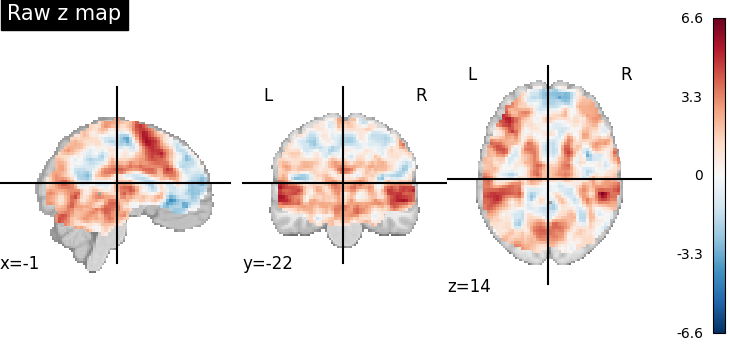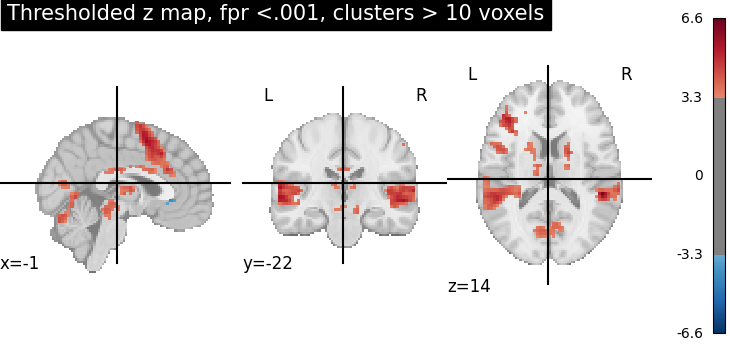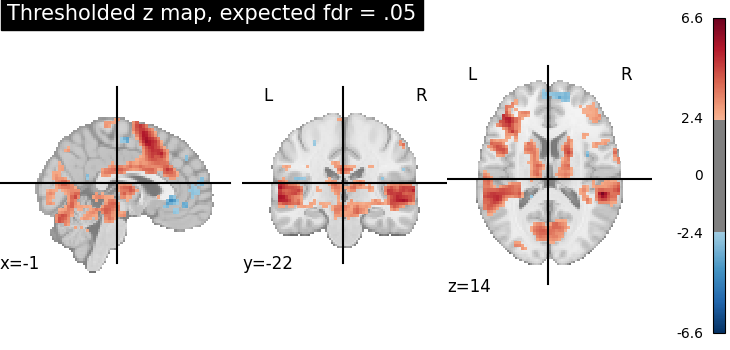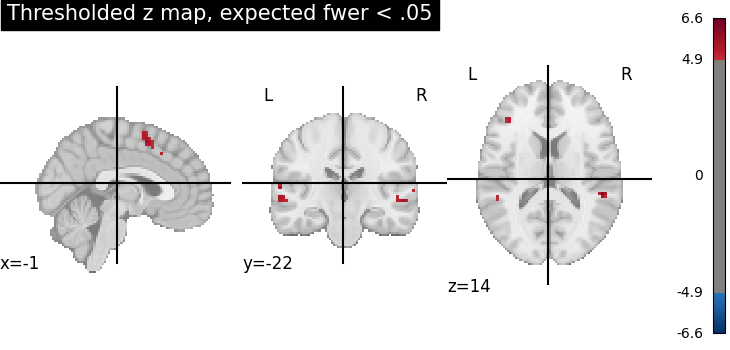# Statistical testing of a second-level analysis#

Perform a one-sample t-test on a bunch of images (a.k.a. second-level analysis in fMRI) and threshold the resulting statistical map.

This example is based on the so-called localizer dataset. It shows activation related to a mental computation task, as opposed to narrative sentence reading/listening.

## Prepare some images for a simple t test#

This is a simple manually performed second level analysis.

```from nilearn import datasets

n_samples = 20
n_subjects=n_samples, legacy_format=False
)
```

Get the set of individual statstical maps (contrast estimates)

```cmap_filenames = localizer_dataset.cmaps
```

## Perform the second level analysis#

First, we define a design matrix for the model. As the model is trivial (one-sample test), the design matrix is just one column with ones.

```import pandas as pd

design_matrix = pd.DataFrame( * n_samples, columns=["intercept"])
```

Next, we specify and estimate the model.

```from nilearn.glm.second_level import SecondLevelModel

second_level_model = SecondLevelModel().fit(
cmap_filenames, design_matrix=design_matrix
)
```

Compute the only possible contrast: the one-sample test. Since there is only one possible contrast, we don’t need to specify it in detail.

```z_map = second_level_model.compute_contrast(output_type="z_score")
```

Threshold the resulting map without multiple comparisons correction, abs(z) > 3.29 (equivalent to p < 0.001), cluster size > 10 voxels.

```from nilearn.image import threshold_img

threshold_img(
z_map,
threshold=3.29,
cluster_threshold=10,
two_sided=True,
)
```
```<nibabel.nifti1.Nifti1Image object at 0x7fd0e94f20a0>
```

This is equivalent to thresholding a z-statistic image with a false positive rate < .001, cluster size > 10 voxels.

```from nilearn.glm import threshold_stats_img

thresholded_map1, threshold1 = threshold_stats_img(
z_map,
alpha=0.001,
height_control="fpr",
cluster_threshold=10,
two_sided=True,
)
```

Now use FDR <.05 (False Discovery Rate) and no cluster-level threshold.

```thresholded_map2, threshold2 = threshold_stats_img(
z_map, alpha=0.05, height_control="fdr"
)
print(f"The FDR=.05 threshold is {threshold2:.3g}")
```
```The FDR=.05 threshold is 2.37
```

Now use FWER <.05 (Family-Wise Error Rate) and no cluster-level threshold. As the data has not been intensively smoothed, we can use a simple Bonferroni correction.

```thresholded_map3, threshold3 = threshold_stats_img(
z_map, alpha=0.05, height_control="bonferroni"
)
print(f"The p<.05 Bonferroni-corrected threshold is {threshold3:.3g}")
```
```The p<.05 Bonferroni-corrected threshold is 4.88
```

## Visualize the results#

First, the unthresholded map.

```from nilearn import plotting

display = plotting.plot_stat_map(z_map, title="Raw z map")
```Second, the p<.001 uncorrected-thresholded map (with only clusters > 10 voxels).

```plotting.plot_stat_map(
thresholded_map1,
cut_coords=display.cut_coords,
threshold=threshold1,
title="Thresholded z map, fpr <.001, clusters > 10 voxels",
)
``````<nilearn.plotting.displays._slicers.OrthoSlicer object at 0x7fd0ea864fd0>
```

Third, the fdr-thresholded map.

```plotting.plot_stat_map(
thresholded_map2,
cut_coords=display.cut_coords,
title="Thresholded z map, expected fdr = .05",
threshold=threshold2,
)
``````<nilearn.plotting.displays._slicers.OrthoSlicer object at 0x7fd0eb36f640>
```

Fourth, the Bonferroni-thresholded map.

```plotting.plot_stat_map(
thresholded_map3,
cut_coords=display.cut_coords,
title="Thresholded z map, expected fwer < .05",
threshold=threshold3,
)
``````<nilearn.plotting.displays._slicers.OrthoSlicer object at 0x7fd0d9e4c910>
```

These different thresholds correspond to different statistical guarantees: in the FWER-corrected image there is only a probability smaller than .05 of observing any false positive voxel. In the FDR-corrected image, 5% of the voxels found are likely to be false positive. In the uncorrected image, one expects a few tens of false positive voxels.

```plotting.show()
```

Total running time of the script: (0 minutes 12.442 seconds)

Estimated memory usage: 9 MB

Gallery generated by Sphinx-Gallery